## Sample Test Problems

1. Derive the commutatorsand. Now show that, that is,raises theeigenvalue but does not change the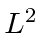eigenvalue.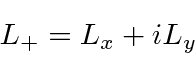Sincecommutes with both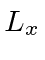and,We have the commutators. Now we apply them to a.So,is also an eigenfunction ofwith the same eigenvalue.does not change.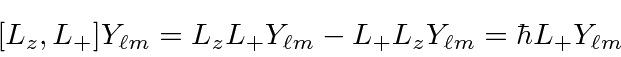So,raises the eigenvalue of.
2. Write the (normalized) state which is an eigenstate ofwith eigenvalueand also an eigenstate ofwith eigenvaluein terms of the usual.
An eigenvalue ofimplies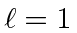. We will need a linear combination of the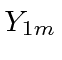to get the eigenstate of.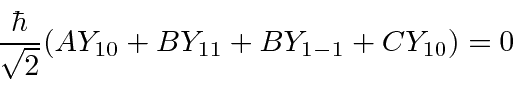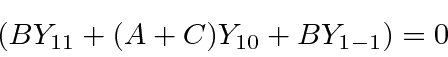Since this is true for all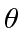and, each term must be zero.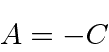The state isThe trivial solution that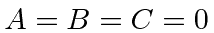is just a zero state, not normalizable to 1.
3. Write the (normalized) state which is an eigenstate ofwith eigenvalueand also an eigenstate ofwith eigenvaluein terms of the usual.
4. Calculate the commutatorsand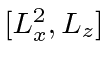.
5. Derive the relation.
6. A particle is in astate and is known to have angular momentum in thedirection equal to. That is. Since we know,must have the form. Find the coefficientsandfornormalized.
7. Calculate the following commutators:,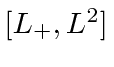,.
8. Prove that, if the Hamiltonian is symmetric under rotations, then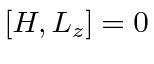.
9. In 3 dimensions, a particle is in the state:whereis some arbitrary radial wave function normalized such that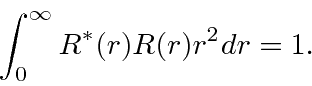a)
Find the value of C that will normalize this wave function.
b)
If a measurement ofis made, what are the possible measured values and what are probabilities for each.
c)
Find the expected value of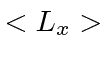in the above state.
10. Two (different) atoms of masses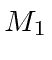andare bound together into the ground state of a diatomic molecule. The binding is such that radial excitations can be neglected at low energy and that the atoms can be assumed to be a constant distanceapart. (We will ignore the small spread around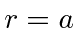.)
a)
What is the energy spectrum due to rotations of the molecule?
b)
Assuming thatis given, write down the energy eigenfunctions for the ground state and the first excited state.
c)
Assuming that both masses are about 1000 MeV, how does the excitation energy of the first excited state compare to thermal energies atK.

Jim Branson 2013-04-22